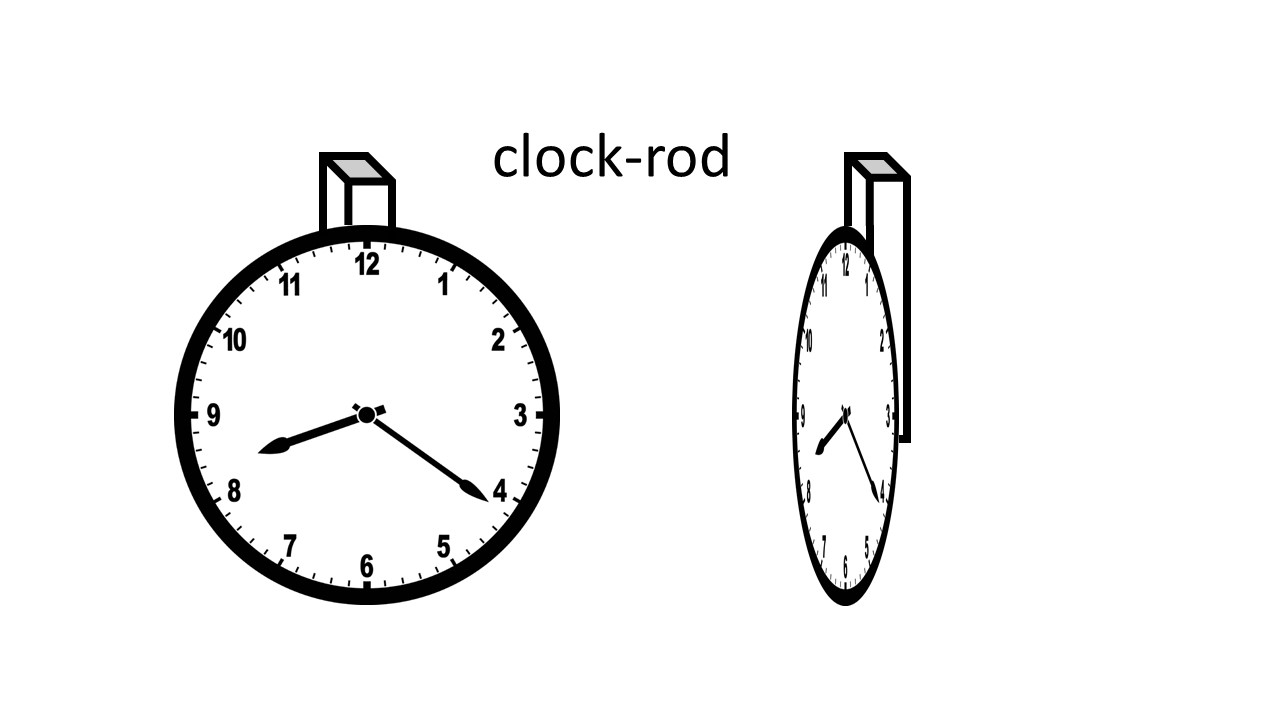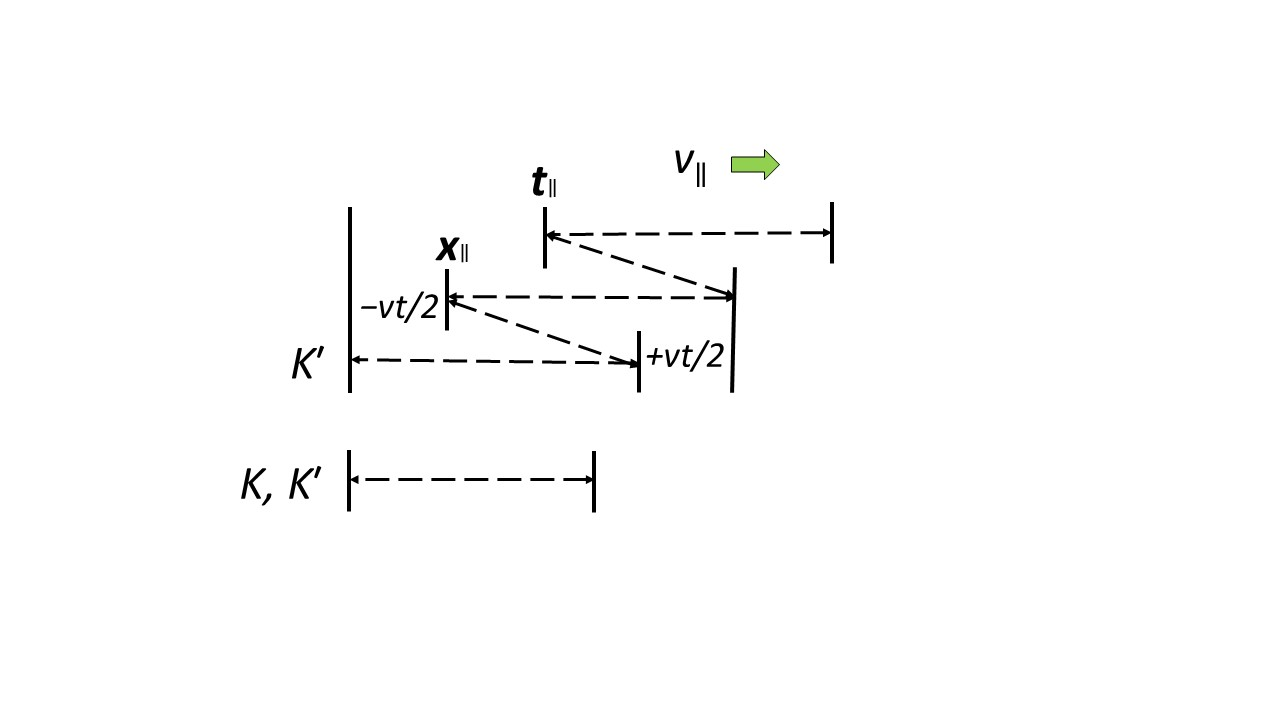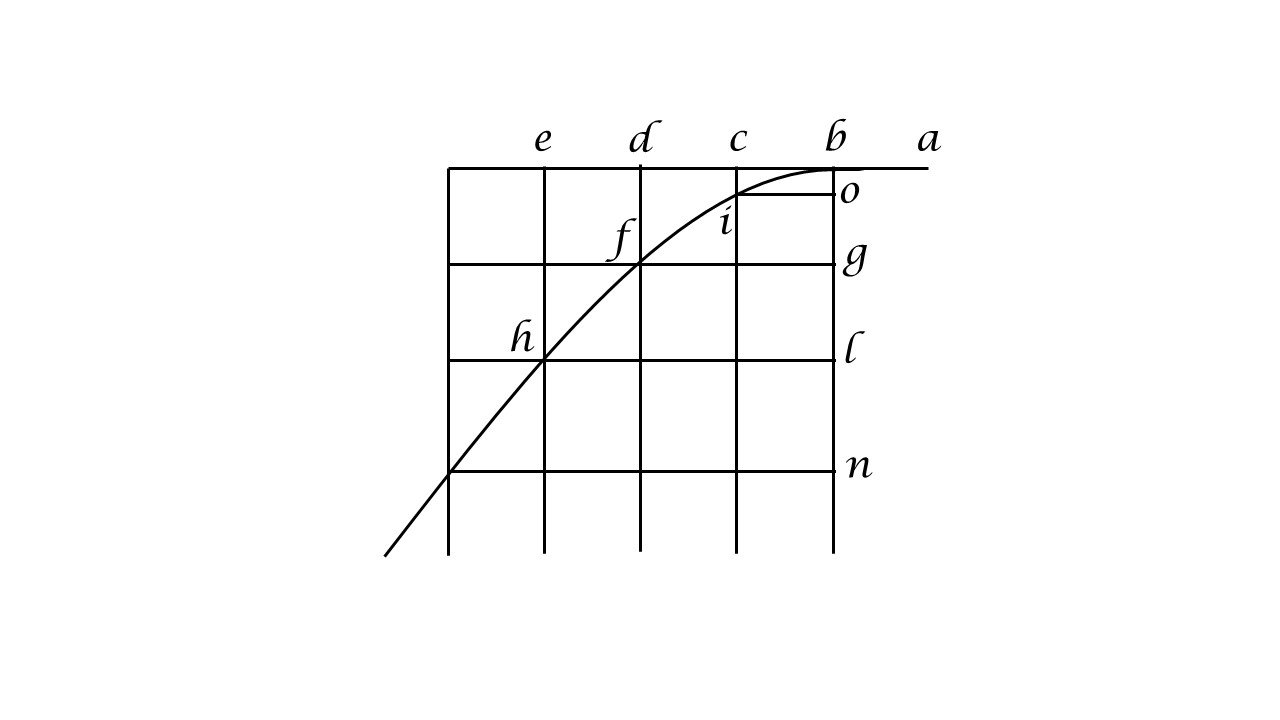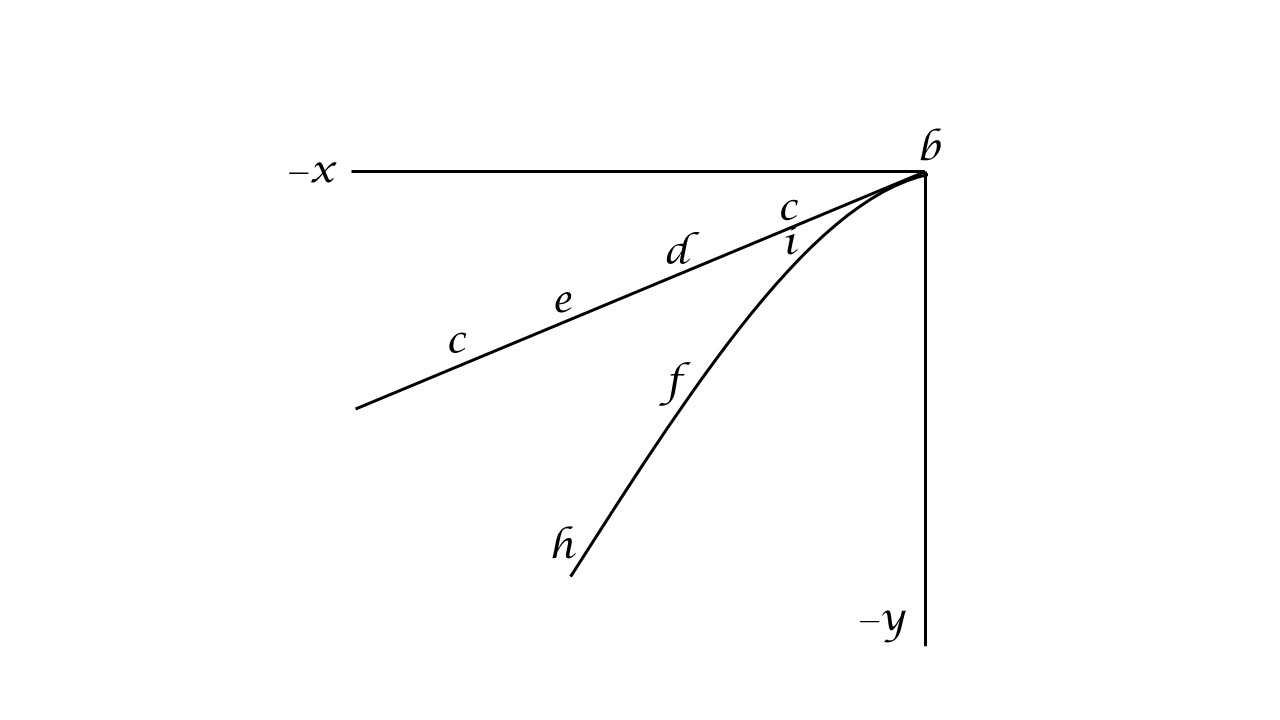iSoul In the beginning is reality.

# Tag Archives: Space & Time

Matters relating to length and duration in physics and transportation

# Clock-rods

A clock-rod is a linear or planar clock with a parallel rod attached to it. A mechanical or electronic clock-rod might look like this:Three clock-rods mutually perpendicular would measure length and duration in all directions.

A light clock-rod is conceptually like this:The clock and rod are parallel to each other so that parallel or perpendicular motion would change either the measurements of either the clock or the rod but not both. A complete harmonic cycle is not affected by motion:The +vt/2 increased distance of the first half-cycle is offset by the −vt/2 decreased distance of the second half-cycle. Likewise for the times +2d/v and −2d/v.

# Kinematic proofs

Displacement with time: displacement s, time t, velocities v1 and v, acceleration a:

To prove: v = v1 + at

a = (vv1) / t    by definition

at = (vv1)     multiply by t

v = v1 + at

To prove: s = v1t + ½ at2

vavg = s / t          by definition

vavg = (v1 + v) / 2        by definition

s / t = (v1 + v) / 2       combining these two

s = (v1 + v) t / 2         multiply by t

s = (v1 + (v1 + at)) t / 2       from above

s = (2v1 + at) t / 2

s = v1t + ½ at2

# Space as time and time as spaceGalileo used the length of uniform motion as a measure of duration, i.e., time (Dialogues Concerning Two New Sciences Tr. by Henry Crew and Alfonso de Salvio, 1914):

Accordingly we see that while the body moves from b to c with uniform speed, it also falls perpendicularly through the distance ci, and at the end of the time-interval bc finds itself at the point i. p.199

Without getting into the details of the Figure 108, notice the shift of language: “the body moves from b to c” [i.e., a length-interval], then “the time-interval bc“. Galileo uses a length interval to measure a time-interval, which is justified since the motion is “with uniform speed”.

Let there be a ball dropped out the window by a passenger on a train in uniform motion. Consider the following four scenarios, in which the length or duration of a uniform motion is measured: (1) looking down above the moving ball, measuring the length of fall; (2) looking down above the moving ball, measuring the (uniform) duration of fall; (3) looking from the side, measuring the length of motion in two dimensions; and (4) looking from the side, measuring the (uniform) duration of motion in two dimensions.

# Metric postulates for time geometry

Geometry was developed by the ancient Greeks in the language of length, but it is an abstraction that may be applied to anything that conforms to its definitions and axioms. Here we apply it to duration. We will use Brossard’s “Metric Postulates for Space Geometry” [American Mathematical Monthly, Vol. 74, No. 7, Aug.-Sep., 1967, pp. 777-788], which generalizes the plane metric geometry of Birkhoff to three dimensions. First, Brossard:

Primitive notions. Points are abstract undefined objects. Primitive terms are: point, distance, line, ray or half-line, half-bundle of rays, and angular measure. The set of all points will be denoted by the letter S and some subsets of S are called lines. The plane as well as the three-dimensional space shall not be taken as primitive terms but will be constructed.

Axioms on points, lines, and distance. The axioms on the points and on the lines are:

E1. There exist at least two points in S.

E2. A line contains at least two points.

E3. Through two distinct points there is one and only one line.

E4. There exist points not all on the same line.

A set of points is said to be collinear if this set is a subset of a line. Two sets are collinear if the union of these sets is collinear. The axioms on distance are:

D1. If A and B are points, then d(AB) is a nonnegative real number.

D2. For points A and B, d(AB) = 0 if and only if A = B.

D3. If A and B are points, then d(AB) = d(BA).

# Length and duration in time and space

Length and duration are defined by their measurement. Length is that which is measured by a rigid rod or its equivalent.

Length is “extension in space” (Dict. of Physics).

Duration is that which is measured by a clock or its equivalent.

Duration is “time measured by a clock or comparable mechanism” (Dict. of Physics).

Time and space and defined as concepts. Time is a local uniform motion that indicates the length or duration of local events by convention.

Time is “The dimension of the physical universe which, at a given place, orders the sequence of events.” (Dict. of Physics).

Space is a three-dimensional expanse whose extent is measured by length and duration.

“Space, a boundless, three-dimensional extent in which objects and events occur and have relative position and direction.” (Encyclopedia Britannica)

The difference between length and duration is the relation between the observed and the observed, the measurand. The question is, which one is the reference quantity and which one is the measured quantity. If the observer is (or has) the reference, and the observed is the measurand, then the value measured is length. If the observer is the measurand, and the observed is (or has) the reference, then the value measured is duration.

Time is derived from (1) three orthogonal uniform motions, or (2) one orthogonal uniform motion in which the three orthogonal uniform motions are components, or (3) the distance from the origin of the one orthogonal uniform motion in (2). In both (1) and (2) time is derived from three-dimensional, whereas in (3) time is one-dimensional or a scalar.

Space is derived from (1) three orthogonal uniform motions, or (2) one orthogonal uniform motion in which the three orthogonal uniform motions are components, or (3) the distance from the origin of the one orthogonal uniform motion in (2). In both (1) and (2) space is derived from three-dimensional, whereas in (3) time is one-dimensional or a scalar.

The difference between time and space is the relation between the observer and observed, or reference and measurand. If the reference motion is at rest in the observer’s frame, then what is measured is the length of motion of an observed body. If the reference motion is at rest in the observed frame, then what is measured is the duration of motion of an observed body.

# Two forms of time and space

The SI metric base unit of length is the metre. The SI base unit of duration is the second. Other units of length are the kilometre, millimetre, inch, foot, mile, etc. Other units of duration are the minute, hour, day (sidereal, solar, etc.), year, etc.

Duration time is also called time. Length time may be called stance. Length space is also called space. Duration space may be called chronotopy.

Time is an independent variable measured in units of length or duration. Space is a dependent geometry of positions measured in units of length or duration.

Time is indicated by a reference uniform motion, which in units of duration is called a clock and in units of length is called a metreloge. The reference rate of uniform motion is a convention that enables one to convert length to duration and vice versa. By appropriate choice of units, this rate may equal one, in which case length and duration may be interchanged in calculations.

In uniform motion the spaces covered are proportional to the time elapsed. Independent space and time are two sides of the same coin.

The independence of time allows it to be selected independent of other variables, as in setting an appointment, a tempo for music, or the duration of a game. Its independence and uniform motion allows time to change by some linear rule.

Have you ever seen a parade? Have you ever been in a parade?A parade is basically a linear (1D) motion. It begins at a point in space and time and ends at a point in space and time. It is planned to progress in a certain order.

The view from the side sees the parade pass by. The parade participants change in time but the location does not. The parade represents the diachronic perspective of motion in time.

The bird’s-eye view from above (as of a camera on a drone) sees the parade as a whole. The time keeps changing but the general location does not. The parade represents the synchronic perspective of motion in time.

The view from a parade participant sees the streetscape pass by. The parade watchers change in space but the chronation does not. The parade represents the diachoric perspective of motion in space.

The view from the plan for the parade sees the parade as a whole. The stance keeps changing but the chronology does not. The parade represents the synchoric perspective of motion in space.

# Independent uniform motion

This continues posts here and here.

The extent of a motion is measured by a reference motion, just as a length is measured by a reference length. The reference motion used to measure other motions is a uniform motion. Galileo’s definition of uniform motion is the following:

By steady or uniform motion, I mean one in which the distances traversed by the moving particle during any equal intervals of time, are themselves equal. [Galileo’s Two New Sciences, Third Day]

Because equality is a symmetric relation, this could also be expressed as follows:

A steady or uniform motion is one in which the travel times of the moving particle during any equal intervals of space, are themselves equal.

Another way of saying this is that for a uniform motion the intervals of space and the corresponding intervals of time are proportional. That is, a uniform rate of motion is constant.

There are two measures of the extent of a motion, length and duration. Applied to the reference motion, these two measures produce two scalars, a measure of length called stance, and a measure of duration called time. Given a reference starting spacepoint, the length since the start point is called the stance, and the duration since the start timepoint is called the time. Because of the proportionality of uniform motion, given knowledge of stance or time along with the uniform rate, one may deduce the other measure.

The reference motion must also be an independent motion, not dependent on other motions, and it must continue indefinitely, so that any other motion would be at some point simultaneous or simulstanceous with the reference motion. Because of this, any motion may be a function of the reference motion.

This independent, uniform reference motion is commonly represented by a clock, which registers uniform motion continually. Even if the clock’s motion is a uniform circular motion, it represents a uniform linear motion as the numbers increase linearly. The reference motion may equally well be a metreloge, which is a uniform motion that registers length continually. As a clock may be a uniform angular motion whose angles register durations, a metreloge may be a uniform angular motion whose arcs register lengths.

Measures of the target motion may then be considered as a function of one of the reference measures, which acts as a parameter of the target motion. Parametric differential equations and geometry may then be used to represent the course of a motion.

# Simultaneity and simulstanceity

Max Jammer’s book Concepts of Simultaneity (Johns Hopkins UP, 2006) describes the significance, meaning, and history of simultaneity in physics. Here are a few excerpts from his Introduction:

… Einstein himself once admitted: “By means of a revision of the concept of simultaneity in a shapable form I arrived at the special relativity theory.” p.3

That not only temporal but also spatial measurements depend on the notion of simultaneity follows from the simple fact that “the length of a moving line-segment is the distance between simultaneous positions of its endpoints,” as Hans Reichenbach … convincingly demonstrated. Having shown that “space measurements are reducible to time measurements” he concluded that “time is therefore logically prior to space.” p. 4-5

P. F. Browne rightly pointed out that all relativistic effects are ultimately “direct consequences of the relativity of simultaneity.” p.5

One might give the dual to the second statement as: That not only spatial but also temporal measurements depend on the notion of simulstanceity follows from the simple fact that “the duration of a moving line-segment is the time interval between simulstanceous chronations of its endpoints. Space is therefore logically prior to time.

In the next chapter, Terminological Preliminaries, Jammer clarifies the relevant concepts. It is ironic that he gives an early example of the metonym “of spatial terms to denote temporal relations that is frequently encountered both in ancient and in modern languages.” (p.9) Space has priority in language.

# Independent variable dimension

This continues the series of posts, see here.

Let’s begin with Galileo’s figure for uniform motion and uniform accelerated motion:Let the horizontal uniform motion be situated in a 2D x, y coordinate system:The dependent uniform acceleration moves in an additional dimension, z, and so has 3D coordinates.

If the independent uniform motion is measured by time, then time has 2D coordinates. The coordinates are proportional to one another, and so may just as well be replaced by a scalar of the signed magnitude. The same can be done if the uniform motion has 3D coordinates. The scalar time is proportional to the common measure of time, with the appropriate rate of motion, it is the same as scalar time.

One can say the same if the independent uniform motion is measured by length, then length has 2D (or 3D) coordinates. This is nothing new, but because of the uniform motion, the coordinate lengths are proportional to one another, and so may just as well be replaced by a single scalar of the signed magnitude. In this way, length becomes a scalar, called here the stance.